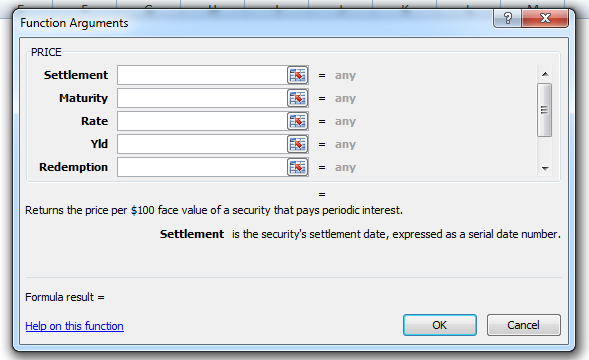# PRICE Function – Calculate Bond Price – Excel & Google Sheet

This tutorial demonstrates how to use the Excel PRICE Function in Excel to calculate the bond price.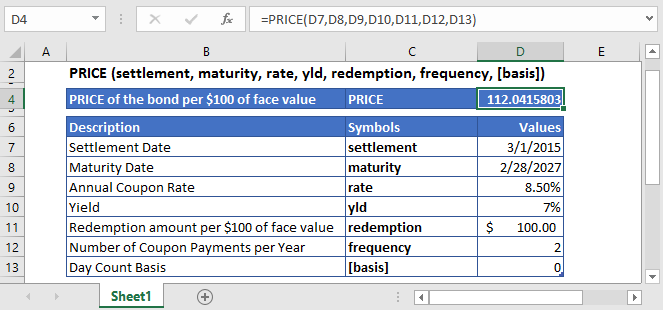## PRICE Function Overview

The PRICE Function Calculates the price of a bond.

To use the PRICE Excel Worksheet Function, select a cell and type: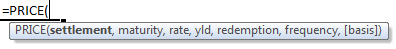(Notice how the formula inputs appear)

### PRICE Function Syntax and Inputs:

settlement – It’s the settlement date of the security or the date at which the security is purchased. It’s the date that comes after the issuing date of the security.

maturity – It’s the date at which the bond or security expires, and the principal amount is paid back to bond or security holder.

rate – It’s the annual interest rate of the bond or security at which the coupon payments are processed.

yld – It’s the annual yield of bond or security.

redemption – The bond’s or security’s redemption value per \$100 face value that is reimbursed to bond or security holder on the redemption date.

frequency – It refers to the number of periodic coupon payments per year. The value of frequency for annual, semi-annual, and quarterly payments are 1, 2, and 4, respectively.

basis – OPTIONAL. It specifies the type of day counting to be used by the security or bond. Possible values can be:

 Basis Day Count 0 US (NASD) 30/360 1 Actual/actual 2 Acutal/360 3 Acutal/365 4 European 30/360

If the basis argument is omitted, it assumes its default value i.e. US (NASD) 30/360.

## What Is PRICE?

It’s the price of the bond. The bond price is the present discounted value of the bond’s future cash flows.

When N > 1 PRICE is calculated using the following equation: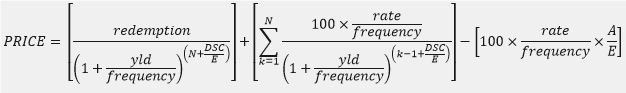And when N = 1 then the PRICE is calculated with the following equation: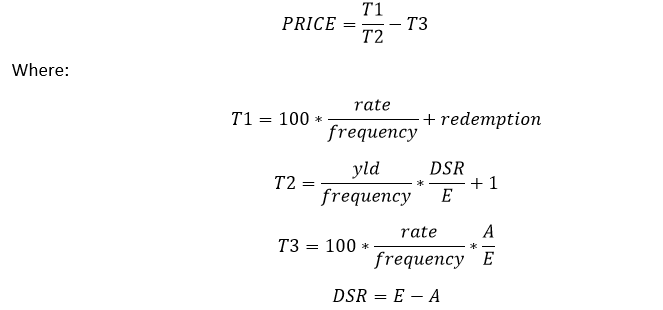DSC = number of days from settlement to next coupon date

E = number of days in coupon period in which the settlement date falls

A = number of days from the beginning of the coupon period to the settlement date.

## What Is Excel PRICE Function?

The Excel PRICE function calculates the price of a bond or security per \$100 face value, which also pays period interest.

### Calculate Price of a corporate bond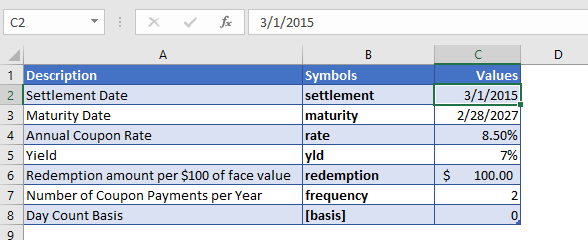Suppose we want to calculate the price per \$100 face value of the corporate bond purchased on March 1, 2015. Other details of the corporate bond are mentioned above in the table.

The Formula used for the calculation of Price of the corporate bond is: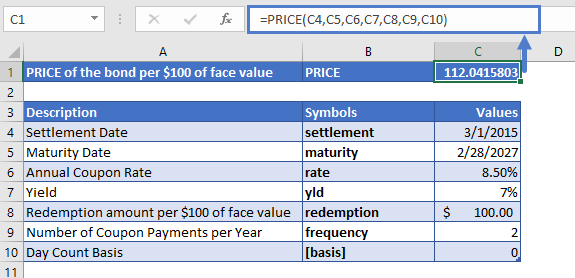The PRICE function returns the value:

PRICE = 112.04

In other words, the Price of the corporate bond per \$100 face value is \$112.04.

### Calculate Price of a fixed-income security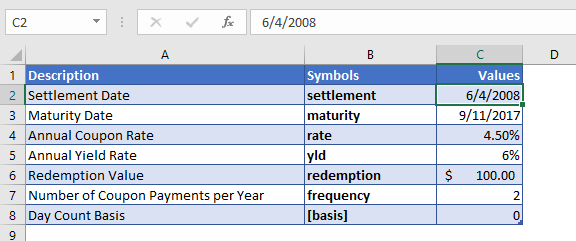Now let’s calculate the price fixed-income security per \$100 of face value. The coupon payments of the security are going to be semi-annually by the default day count basis. Other details of the fixed-income security are mentioned in the above table.

The Formula used for the calculation of Price of the fixed-income security is: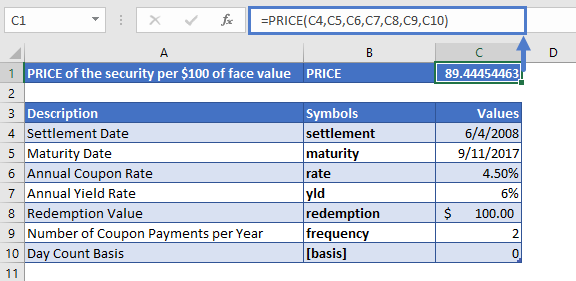The PRICE function returns the following price of the fixed-income security per \$100 face value of a security that pays periodic interest

PRICE = \$89.44

All of the above examples work exactly the same in Google Sheets as in Excel.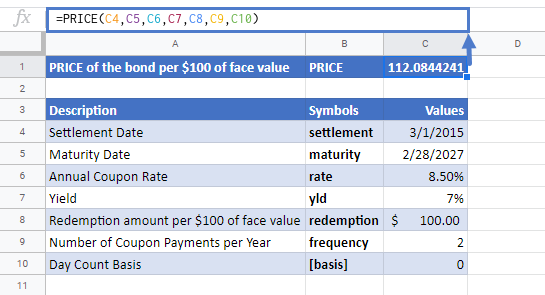Use the PRICE Function to calculate the price of a bond or similar security.

#NUM! Error occurs if the settlement date is greater or equal than the maturity date; or the values of the rate, yld, redemption, frequency, or [basis] arguments are not valid numbers (i.e. rate < 0; or yld < 0; or redemption ≤ 0; or frequency is any value other than 1, 2 or 4; or [basis] value is other than 0, 1, 2, 3, or 4)

#VALUE! Error occurs if the dates of the settlement or the maturity arguments are not valid Excel dates.

It is recommended that the settlement and maturity dates should be entered in the YIELD function as references to cells containing the dates or dates returned from formulas.

## PRICE Examples in VBAYou can also use the PRICE function in VBA. Type:
`Application.Worksheetfunction.Price(settlement,maturity,rate,yld,redemption,FREQUENCY,basis)`
For the function arguments (settlement, etc.), you can either enter them directly into the function or define variables to use instead.

`=AND(`